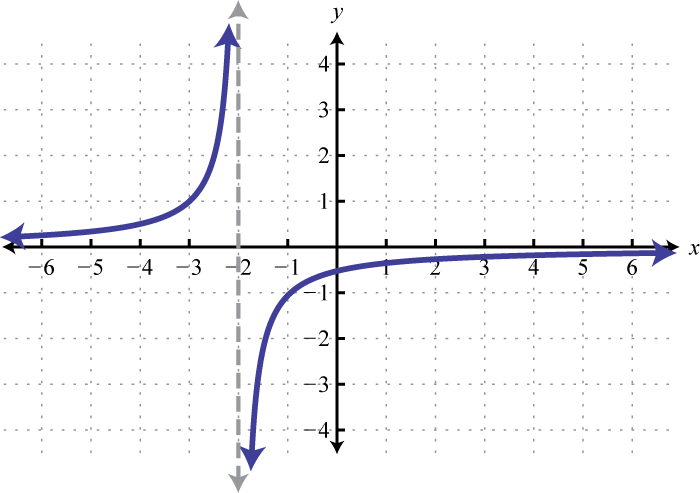This is “Using Transformations to Graph Functions”, section 2.5 from the book Advanced Algebra (v. 1.0). For details on it (including licensing), click here.

Has this book helped you? Consider passing it on:
Creative Commons supports free culture from music to education. Their licenses helped make this book available to you.
DonorsChoose.org helps people like you help teachers fund their classroom projects, from art supplies to books to calculators.

## 2.5 Using Transformations to Graph Functions

### Learning Objectives

1. Define the rigid transformations and use them to sketch graphs.
2. Define the non-rigid transformations and use them to sketch graphs.

## Vertical and Horizontal Translations

When the graph of a function is changed in appearance and/or location we call it a transformation. There are two types of transformations. A rigid transformationA set of operations that change the location of a graph in a coordinate plane but leave the size and shape unchanged. changes the location of the function in a coordinate plane, but leaves the size and shape of the graph unchanged. A non-rigid transformationA set of operations that change the size and/or shape of a graph in a coordinate plane. changes the size and/or shape of the graph.

A vertical translationA rigid transformation that shifts a graph up or down. is a rigid transformation that shifts a graph up or down relative to the original graph. This occurs when a constant is added to any function. If we add a positive constant to each y-coordinate, the graph will shift up. If we add a negative constant, the graph will shift down. For example, consider the functions $g(x)=x2−3$ and $h(x)=x2+3.$ Begin by evaluating for some values of the independent variable x.Now plot the points and compare the graphs of the functions g and h to the basic graph of $f(x)=x2$, which is shown using a dashed grey curve below.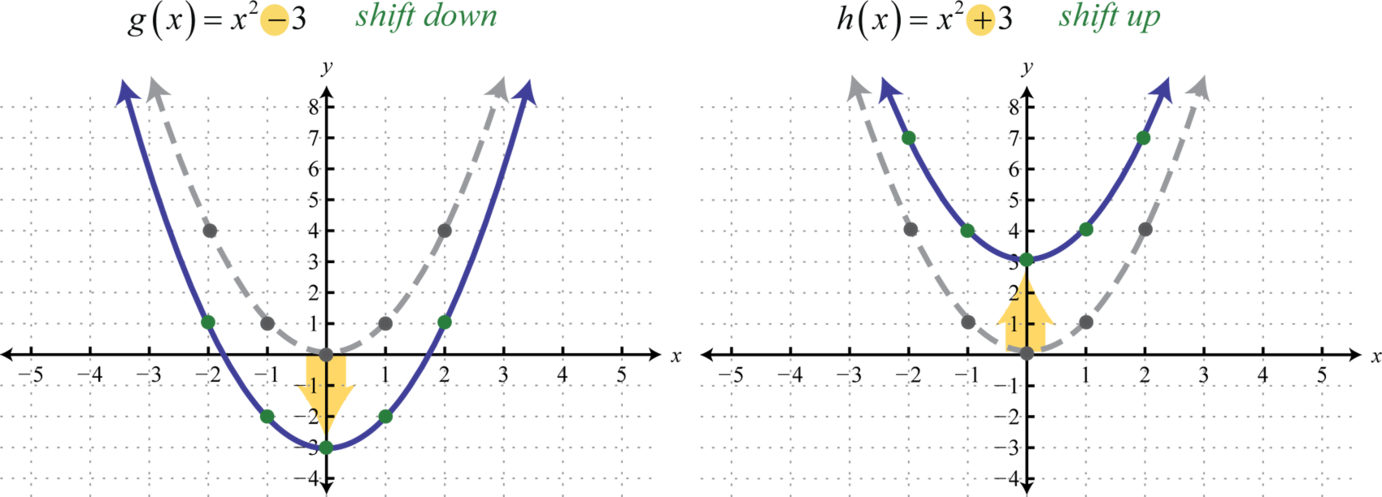The function g shifts the basic graph down 3 units and the function h shifts the basic graph up 3 units. In general, this describes the vertical translations; if k is any positive real number:

 Vertical shift up k units: $F(x)=f(x)+k$ Vertical shift down k units: $F(x)=f(x)−k$

### Example 1

Sketch the graph of $g(x)=x+4.$

Solution:

Begin with the basic function defined by $f(x)=x$ and shift the graph up 4 units.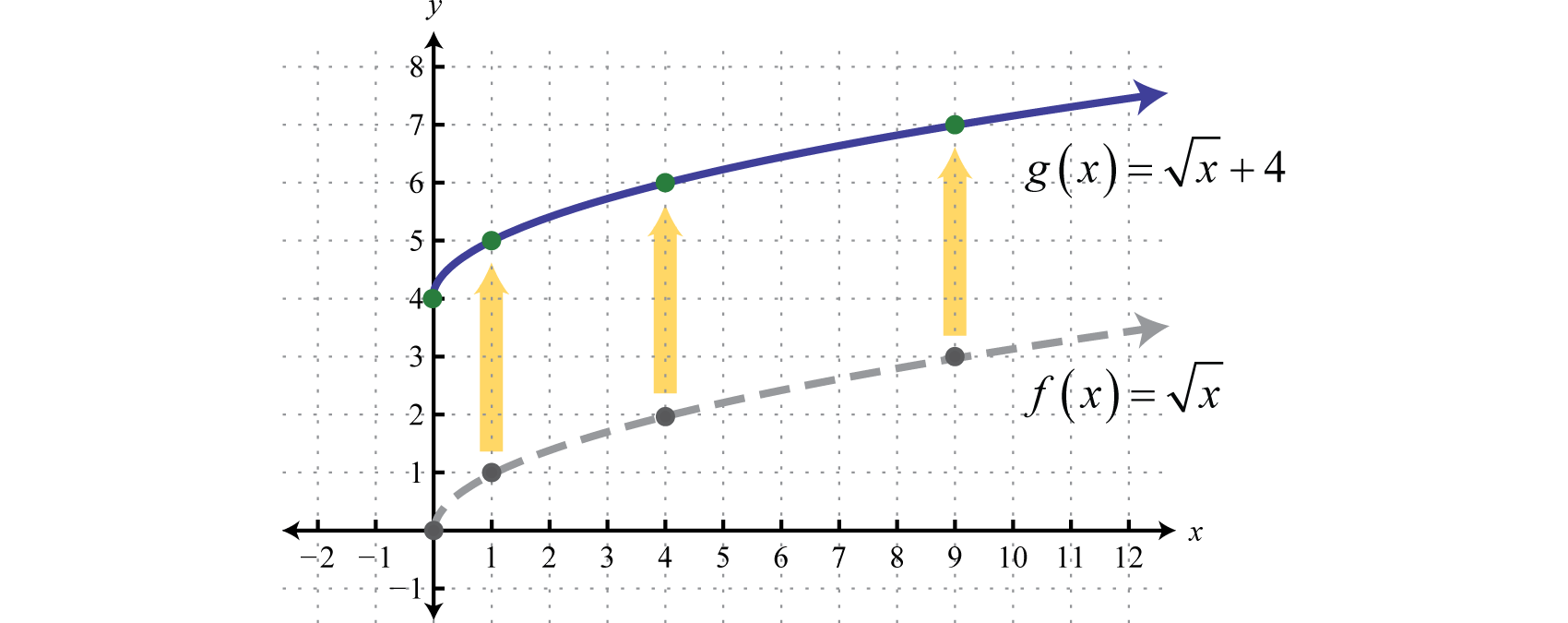A horizontal translationA rigid transformation that shifts a graph left or right. is a rigid transformation that shifts a graph left or right relative to the original graph. This occurs when we add or subtract constants from the x-coordinate before the function is applied. For example, consider the functions defined by $g(x)=(x+3)2$ and $h(x)=(x−3)2$ and create the following tables: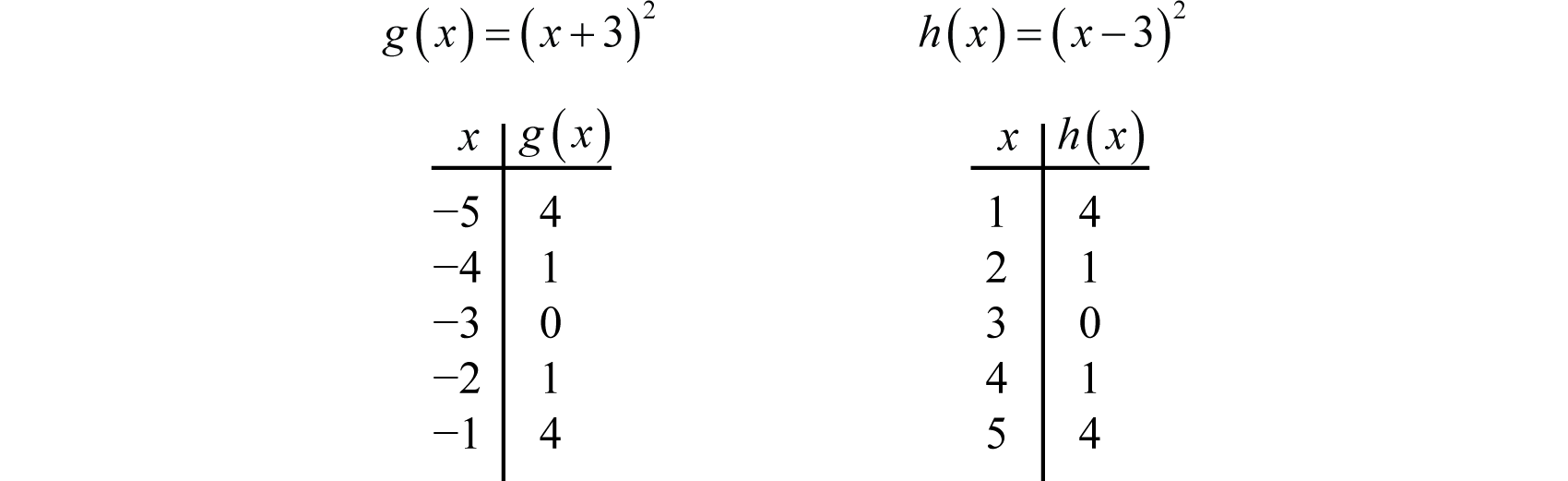Here we add and subtract from the x-coordinates and then square the result. This produces a horizontal translation.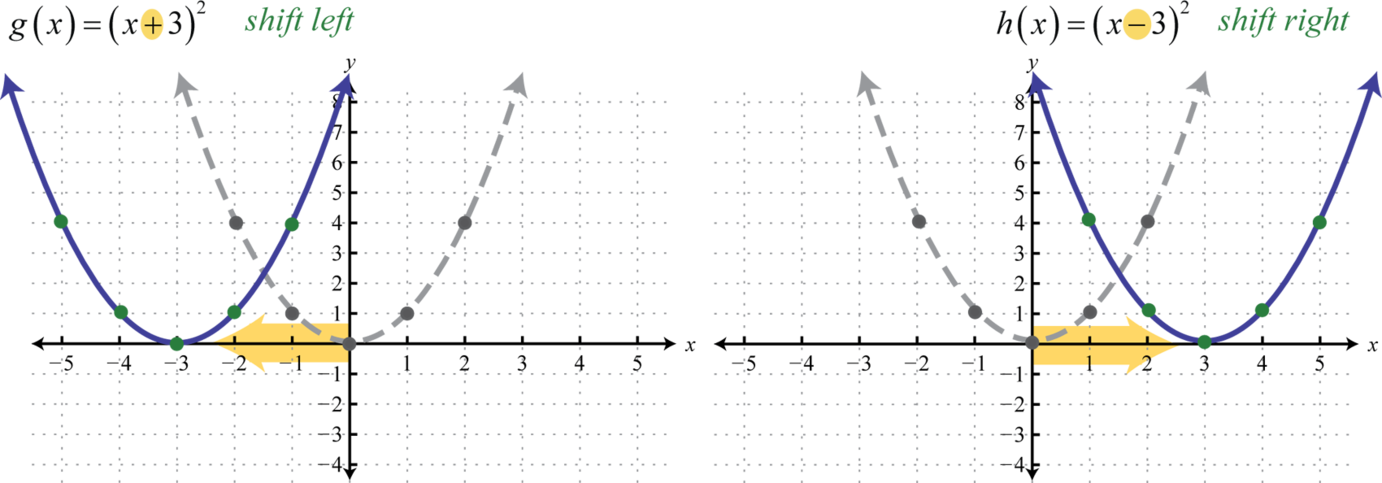Note that this is the opposite of what you might expect. In general, this describes the horizontal translations; if h is any positive real number:

 Horizontal shift left h units: $F(x)=f(x+h)$ Horizontal shift right h units: $F(x)=f(x−h)$

### Example 2

Sketch the graph of $g(x)=(x−4)3.$

Solution:

Begin with a basic cubing function defined by $f(x)=x3$ and shift the graph 4 units to the right.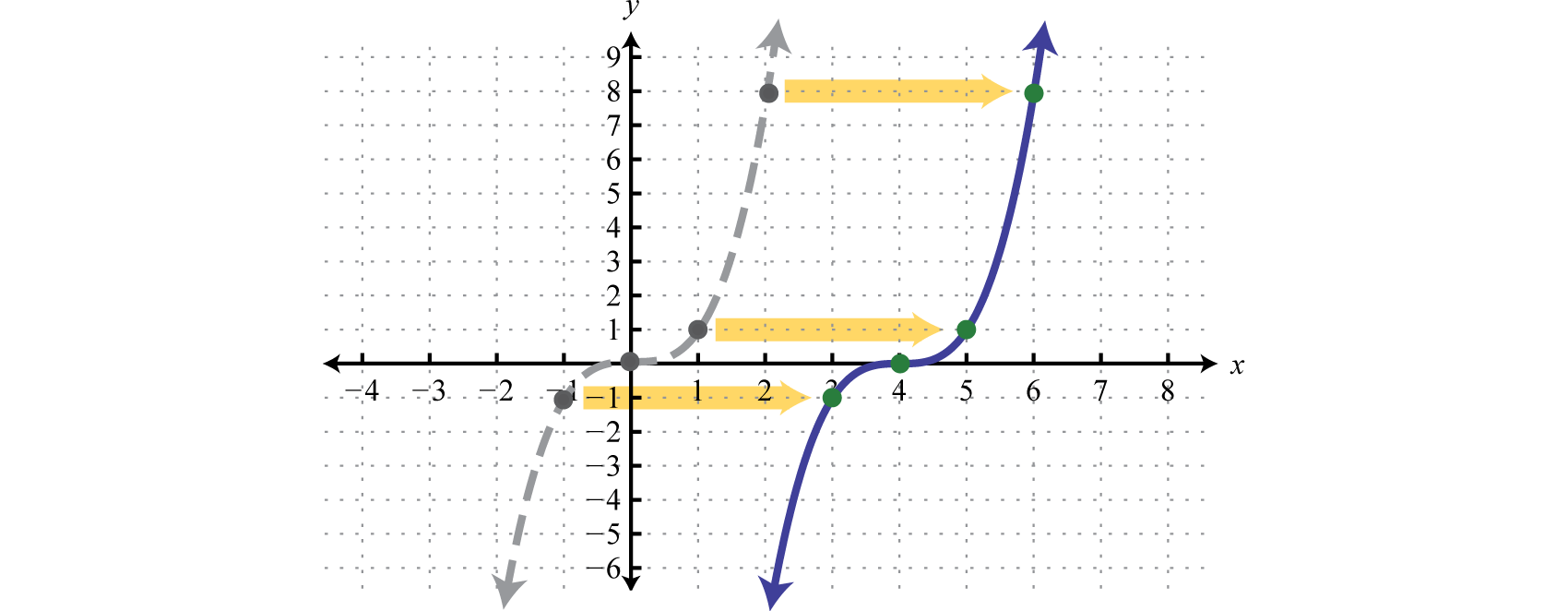It is often the case that combinations of translations occur.

### Example 3

Sketch the graph of $g(x)=|x+3|−5.$

Solution:

$y=|x|Basic functiony=|x+3|Horizontal shift left 3 unitsy=|x+3|−5Vertical shift down 5 units$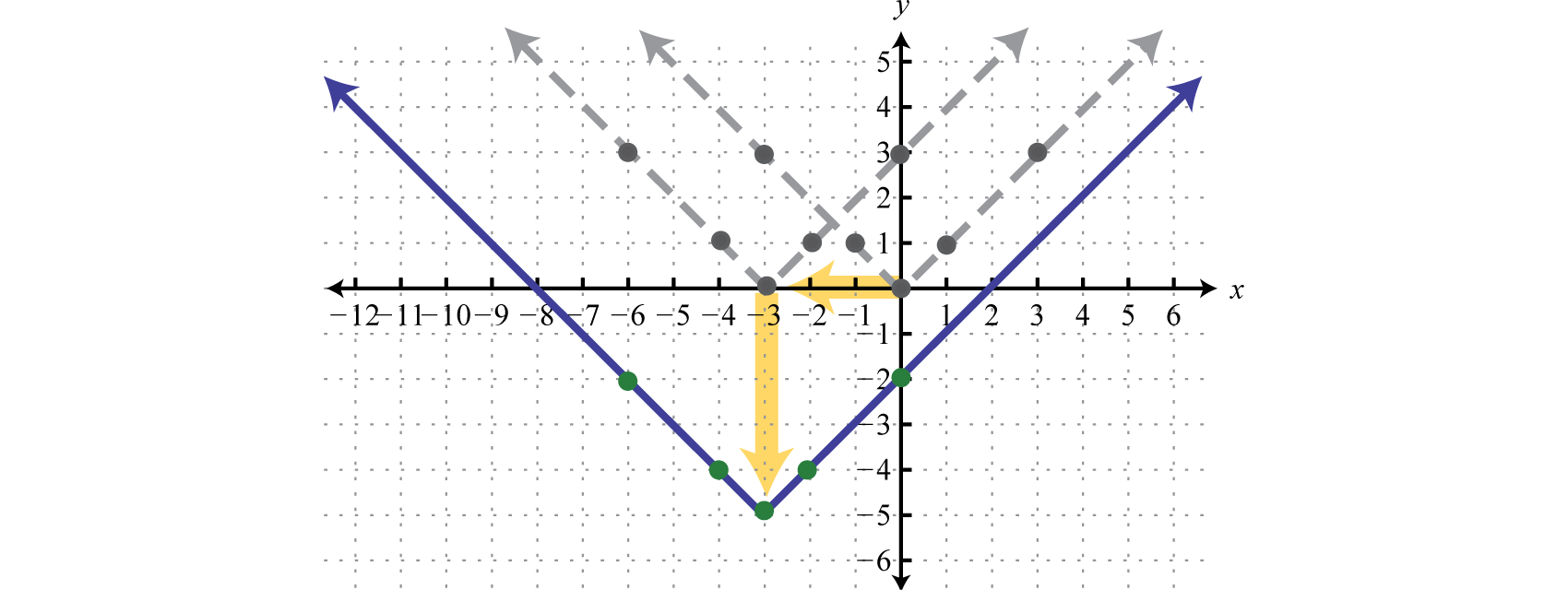The order in which we apply horizontal and vertical translations does not affect the final graph.

### Example 4

Sketch the graph of $g(x)=1x−5+3.$

Solution:

Begin with the reciprocal function and identify the translations.

$y=1xBasic functiony=1x−5Horizontal shift right 5 unitsy=1x−5+3Vertical shift up 3 units$

Take care to shift the vertical asymptote from the y-axis 5 units to the right and shift the horizontal asymptote from the x-axis up 3 units.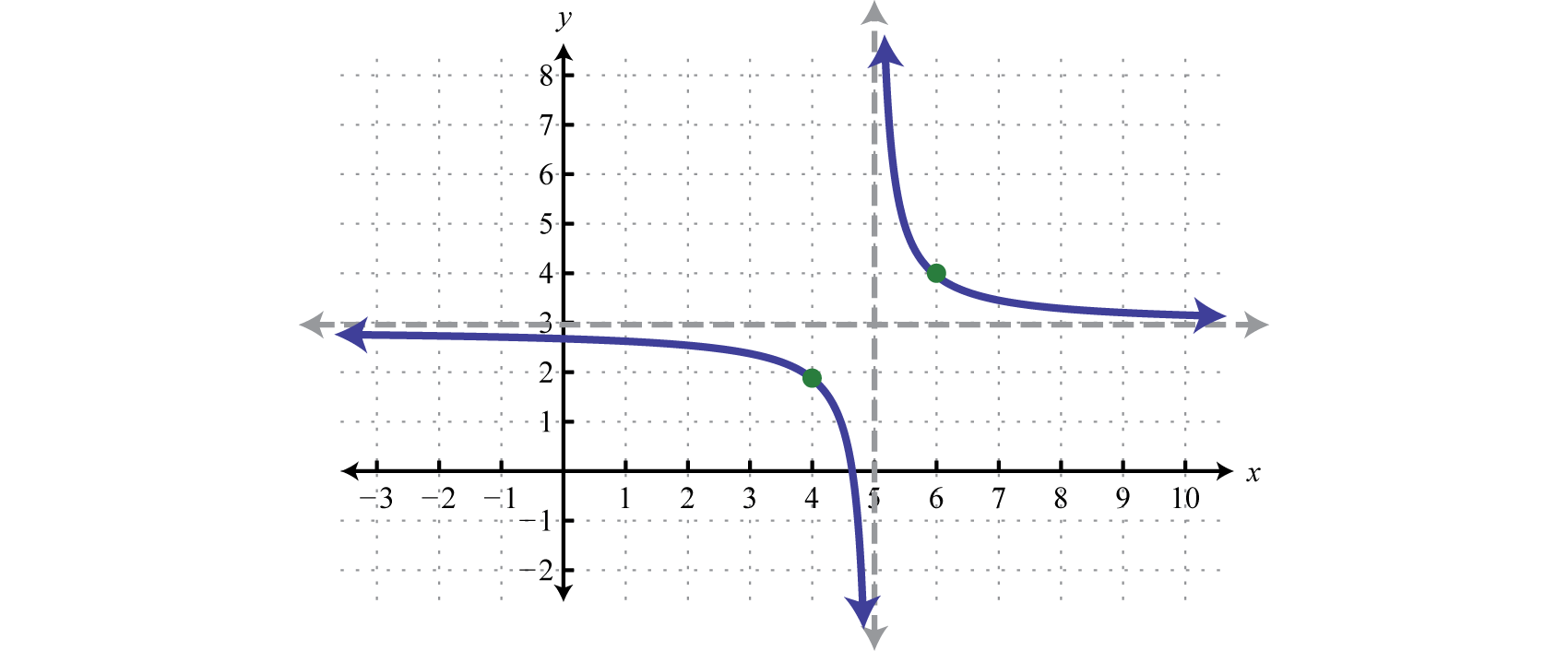Try this! Sketch the graph of $g(x)=(x−2)2+1.$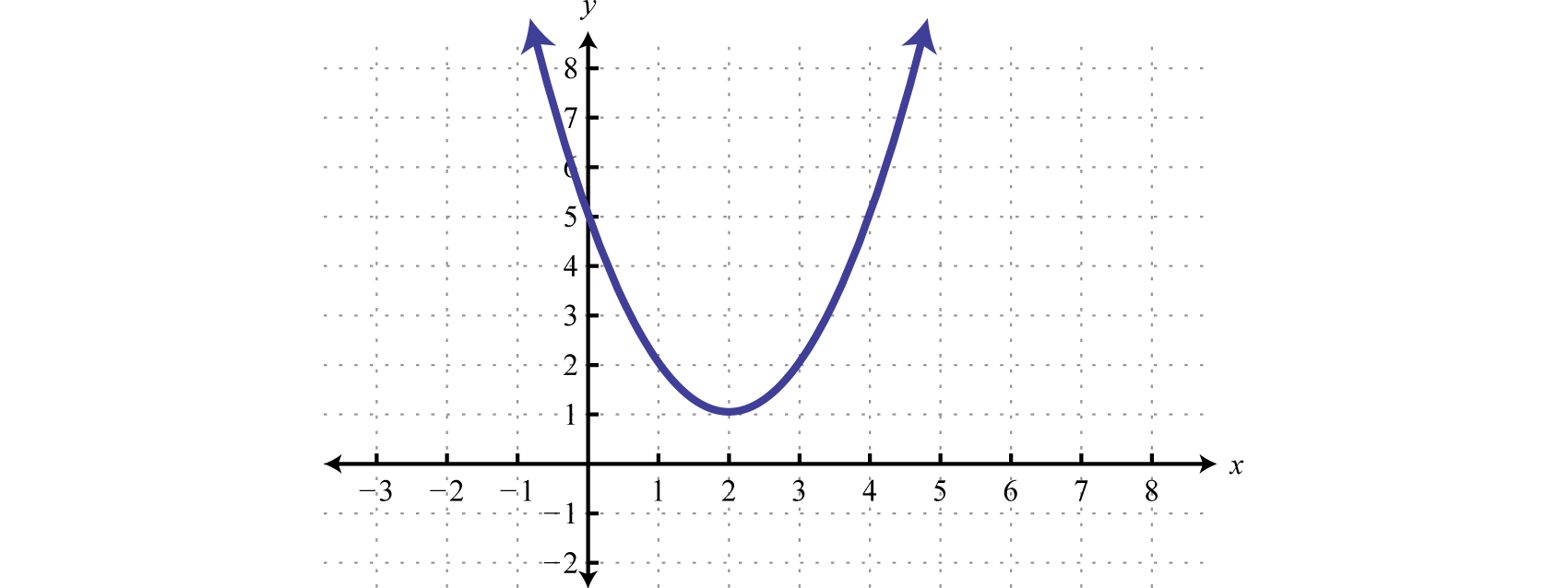## Reflections

A reflectionA transformation that produces a mirror image of the graph about an axis. is a transformation in which a mirror image of the graph is produced about an axis. In this section, we will consider reflections about the x- and y-axis. The graph of a function is reflected about the x-axis if each y-coordinate is multiplied by −1. The graph of a function is reflected about the y-axis if each x-coordinate is multiplied by −1 before the function is applied. For example, consider $g(x)=−x$ and $h(x)=−x.$Compare the graph of g and h to the basic square root function defined by $f(x)=x$, shown dashed in grey below: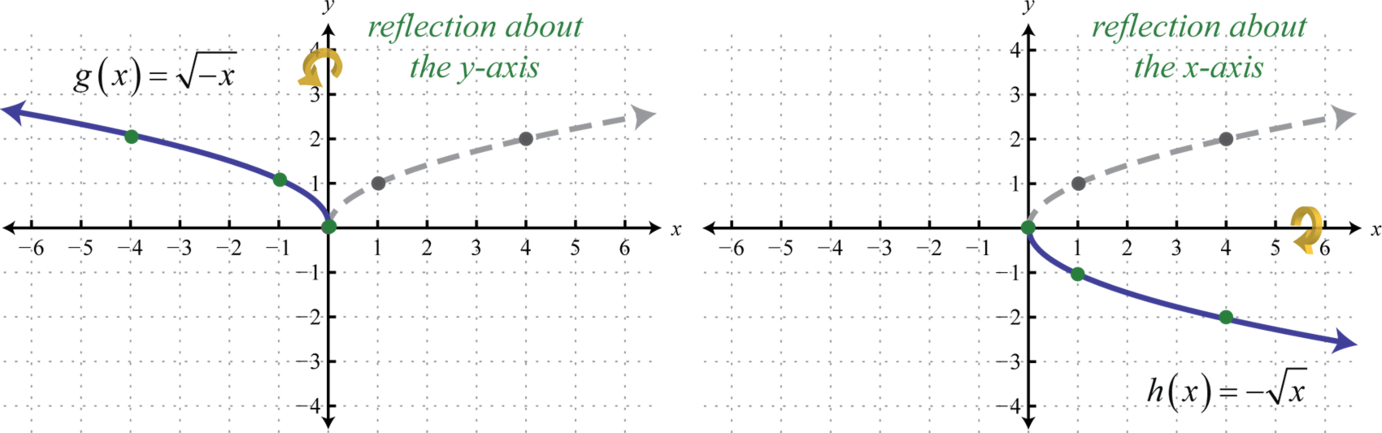The first function g has a negative factor that appears “inside” the function; this produces a reflection about the y-axis. The second function h has a negative factor that appears “outside” the function; this produces a reflection about the x-axis. In general, it is true that:

 Reflection about the y-axis: $F(x)=f(−x)$ Reflection about the x-axis: $F(x)=−f(x)$

When sketching graphs that involve a reflection, consider the reflection first and then apply the vertical and/or horizontal translations.

### Example 5

Sketch the graph of $g(x)=−(x+5)2+3.$

Solution:

Begin with the squaring function and then identify the transformations starting with any reflections.

$y=x2Basic function.y=−x2Reflection about the x-axis.y=−(x+5)2Horizontal shift left 5 units.y=−(x+5)2+3Vertical shift up 3 units.$

Use these translations to sketch the graph.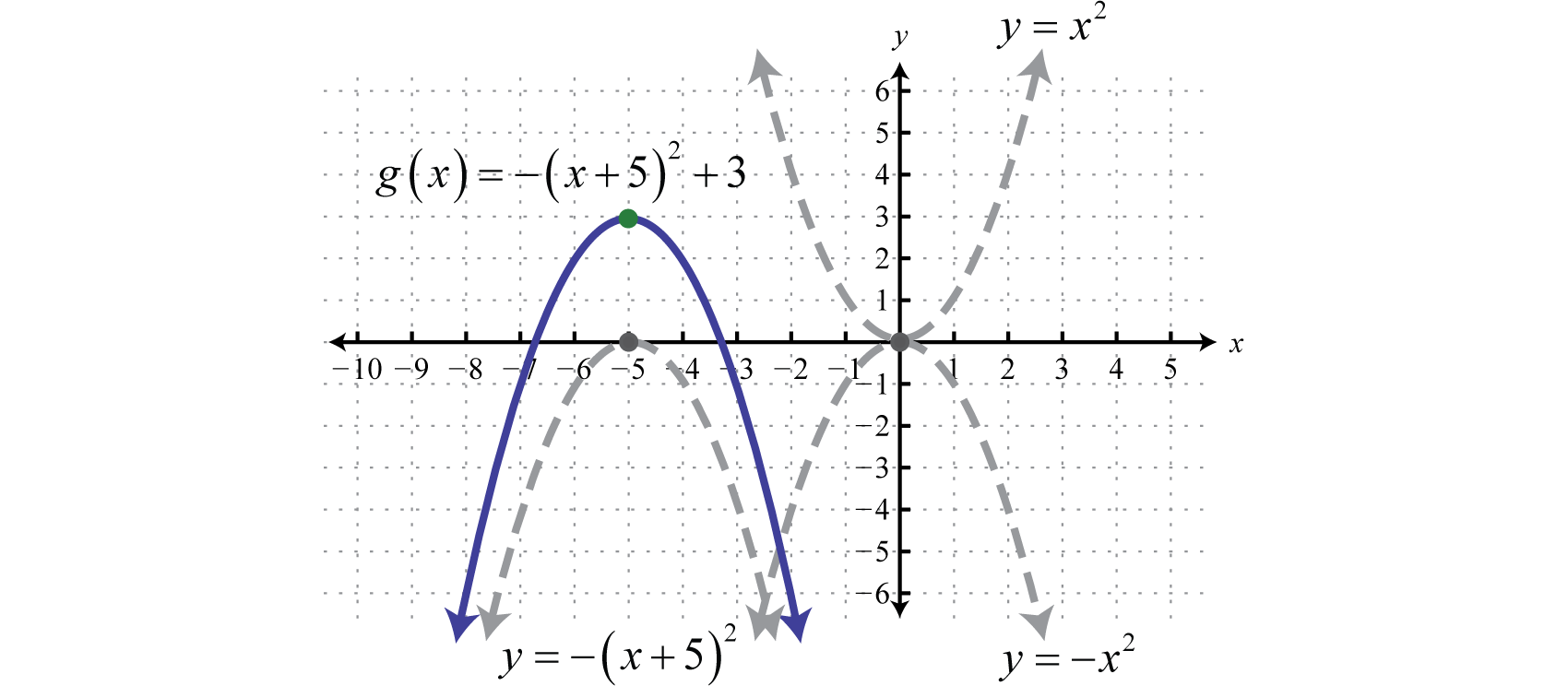Try this! Sketch the graph of $g(x)=−|x|+3.$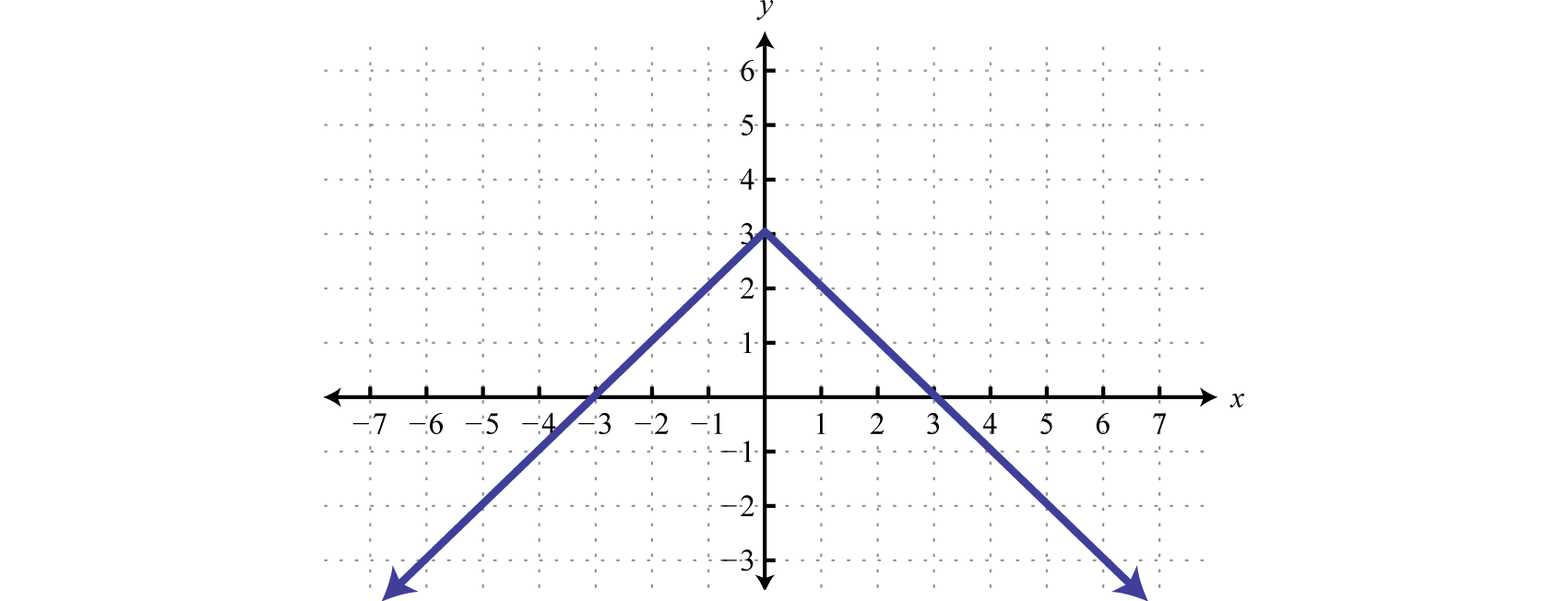## Dilations

Horizontal and vertical translations, as well as reflections, are called rigid transformations because the shape of the basic graph is left unchanged, or rigid. Functions that are multiplied by a real number other than 1, depending on the real number, appear to be stretched vertically or stretched horizontally. This type of non-rigid transformation is called a dilationA non-rigid transformation, produced by multiplying functions by a nonzero real number, which appears to stretch the graph either vertically or horizontally.. For example, we can multiply the squaring function $f(x)=x2$ by 4 and $14$ to see what happens to the graph.Compare the graph of g and h to the basic squaring function defined by $f(x)=x2$, shown dashed in grey below: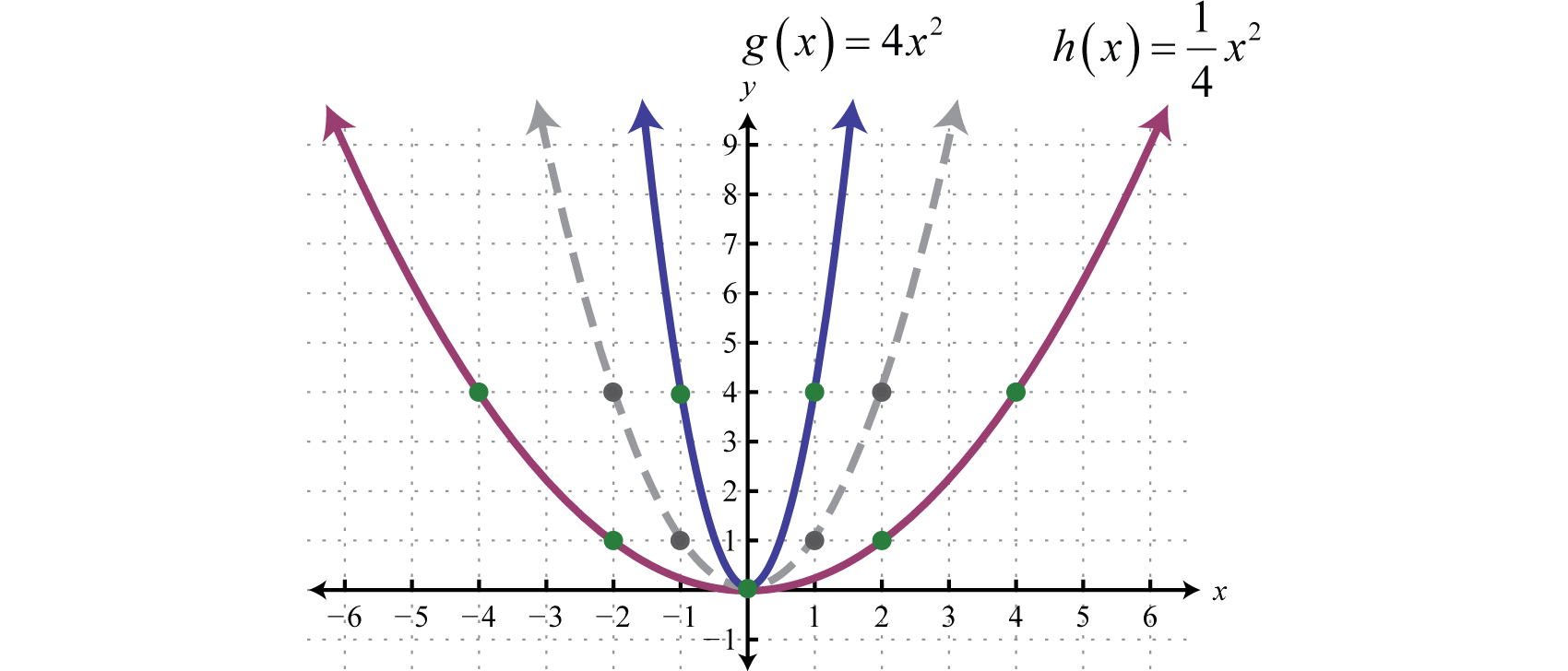The function g is steeper than the basic squaring function and its graph appears to have been stretched vertically. The function h is not as steep as the basic squaring function and appears to have been stretched horizontally.

In general, we have:

 Dilation: $F(x)=a⋅f(x)$

If the factor a is a nonzero fraction between −1 and 1, it will stretch the graph horizontally. Otherwise, the graph will be stretched vertically. If the factor a is negative, then it will produce a reflection as well.

### Example 6

Sketch the graph of $g(x)=−2|x−5|−3.$

Solution:

Here we begin with the product of −2 and the basic absolute value function: $y=−2|x|.$ This results in a reflection and a dilation.

$xyy=−2|x| ←Dilation and reflection−1−2y=−2|−1|=−2⋅1=−200y=−2|0|=−2⋅0=01−2y=−2|1|=−2⋅1=−2$

Use the points {(−1, −2), (0, 0), (1, −2)} to graph the reflected and dilated function $y=−2|x|.$ Then translate this graph 5 units to the right and 3 units down.

$y=−2|x|Basic graph with dilation andreflection about the x−axis.y=−2|x−5|Shift right 5 units.y=−2|x−5|−3Shift down 3 units.$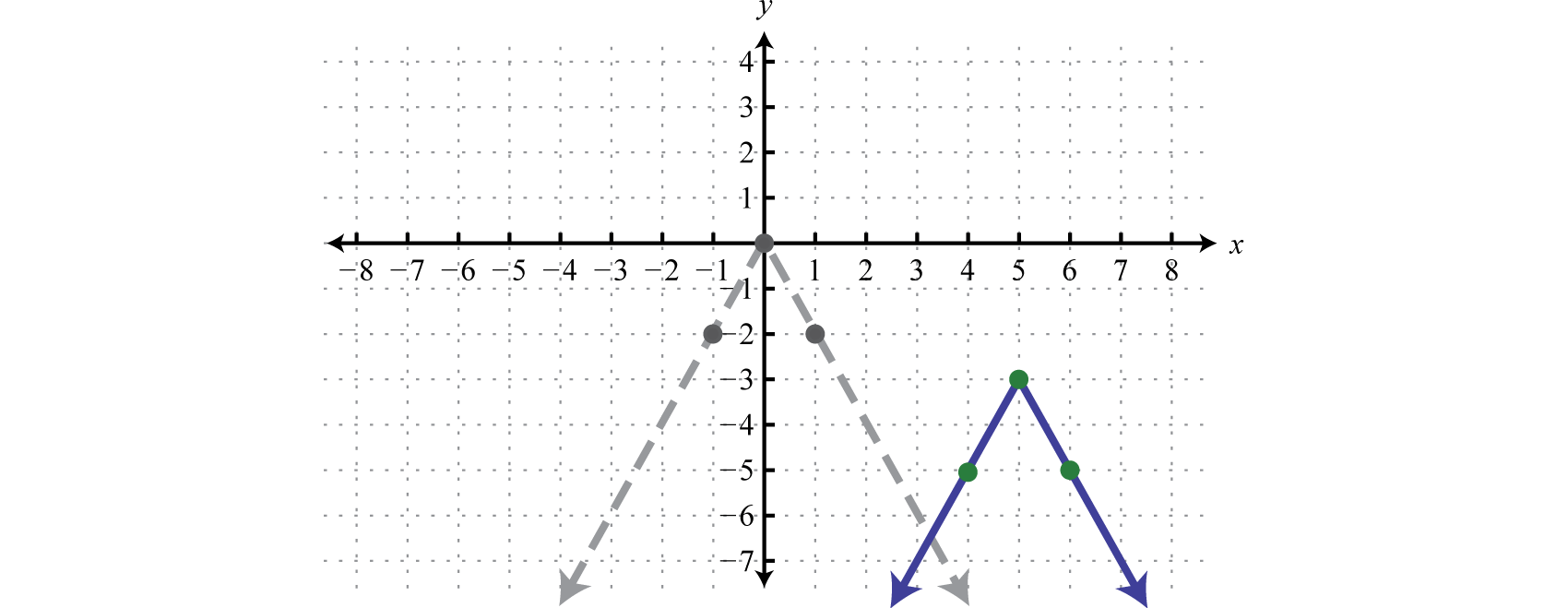In summary, given positive real numbers h and k:

 Vertical shift up k units: $F(x)=f(x)+k$ Vertical shift down k units: $F(x)=f(x)−k$

 Horizontal shift left h units: $F(x)=f(x+h)$ Horizontal shift right h units: $F(x)=f(x−h)$

 Reflection about the y-axis: $F(x)=f(−x)$ Reflection about the x-axis: $F(x)=−f(x)$

 Dilation: $F(x)=a⋅f(x)$

### Key Takeaways

• Identifying transformations allows us to quickly sketch the graph of functions. This skill will be useful as we progress in our study of mathematics. Often a geometric understanding of a problem will lead to a more elegant solution.
• If a positive constant is added to a function, $f(x)+k$, the graph will shift up. If a positive constant is subtracted from a function, $f(x)−k$, the graph will shift down. The basic shape of the graph will remain the same.
• If a positive constant is added to the value in the domain before the function is applied, $f(x+h)$, the graph will shift to the left. If a positive constant is subtracted from the value in the domain before the function is applied, $f(x−h)$, the graph will shift right. The basic shape will remain the same.
• Multiplying a function by a negative constant, $−f(x)$, reflects its graph in the x-axis. Multiplying the values in the domain by −1 before applying the function, $f(−x)$, reflects the graph about the y-axis.
• When applying multiple transformations, apply reflections first.
• Multiplying a function by a constant other than 1, $a⋅f(x)$, produces a dilation. If the constant is a positive number greater than 1, the graph will appear to stretch vertically. If the positive constant is a fraction less than 1, the graph will appear to stretch horizontally.

### Part A: Vertical and Horizontal Translations

Match the graph to the function definition.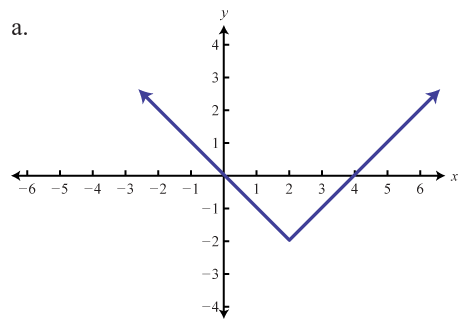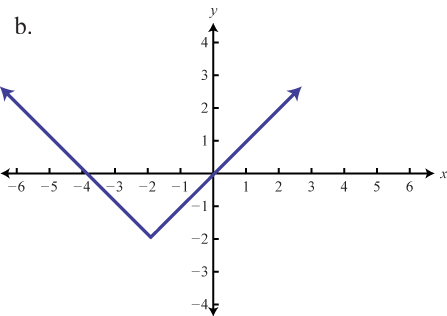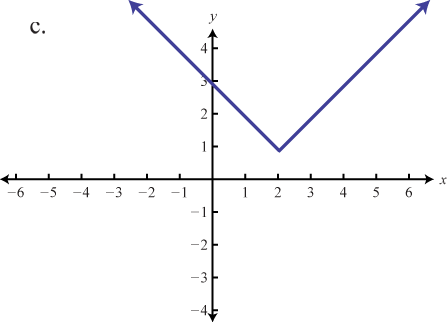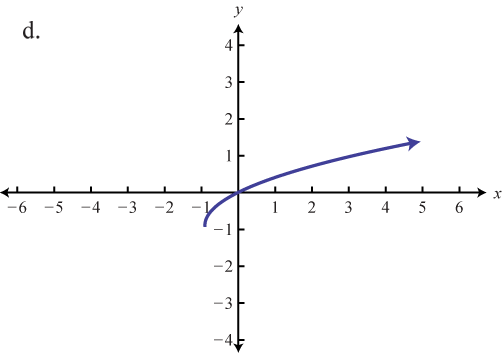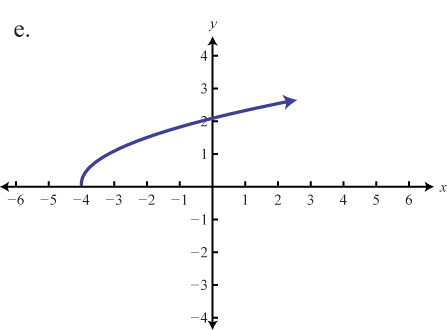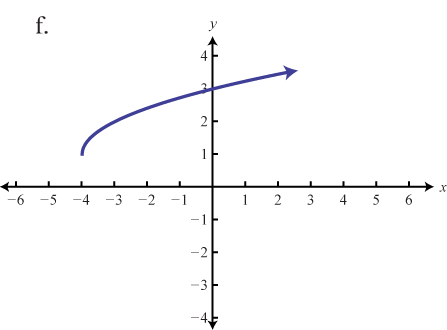1. $f(x)=x+4$

2. $f(x)=|x−2|−2$

3. $f(x)=x+1−1$

4. $f(x)=|x−2|+1$

5. $f(x)=x+4+1$

6. $f(x)=|x+2|−2$

Graph the given function. Identify the basic function and translations used to sketch the graph. Then state the domain and range.

1. $f(x)=x+3$

2. $f(x)=x−2$

3. $g(x)=x2+1$

4. $g(x)=x2−4$

5. $g(x)=(x−5)2$

6. $g(x)=(x+1)2$

7. $g(x)=(x−5)2+2$

8. $g(x)=(x+2)2−5$

9. $h(x)=|x+4|$

10. $h(x)=|x−4|$

11. $h(x)=|x−1|−3$

12. $h(x)=|x+2|−5$

13. $g(x)=x−5$

14. $g(x)=x−5$

15. $g(x)=x−2+1$

16. $g(x)=x+2+3$

17. $h(x)=(x−2)3$

18. $h(x)=x3+4$

19. $h(x)=(x−1)3−4$

20. $h(x)=(x+1)3+3$

21. $f(x)=1x−2$

22. $f(x)=1x+3$

23. $f(x)=1x+5$

24. $f(x)=1x−3$

25. $f(x)=1x+1−2$

26. $f(x)=1x−3+3$

27. $g(x)=−4$

28. $g(x)=2$

29. $f(x)=x−23+6$

30. $f(x)=x+83−4$

Graph the piecewise functions.

1. $h(x)={x2+2 if x<0x+2 if x≥0$
2. $h(x)={x2−3 if x<0x−3 if x≥0$
3. $h(x)={x3−1 if x<0|x−3|−4 if x≥0$
4. $h(x)={ x3ifx<0(x−1)2−1ifx≥0$
5. $h(x)={x2−1 if x<02 if x≥0$
6. $h(x)={x+2ifx<0(x−2)2ifx≥0$
7. $h(x)={(x+10)2−4 if x<−8x+4 if −8≤x<−4x+4 if x≥−4$
8. $f(x)={x+10 if x≤−10|x−5|−15 if −1020$

Write an equation that represents the function whose graph is given.

1.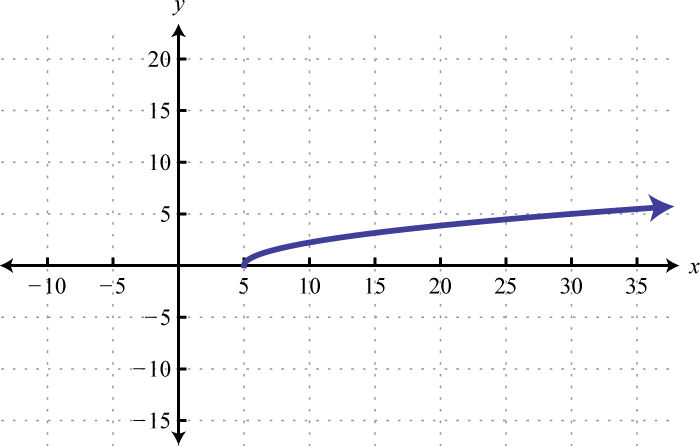2.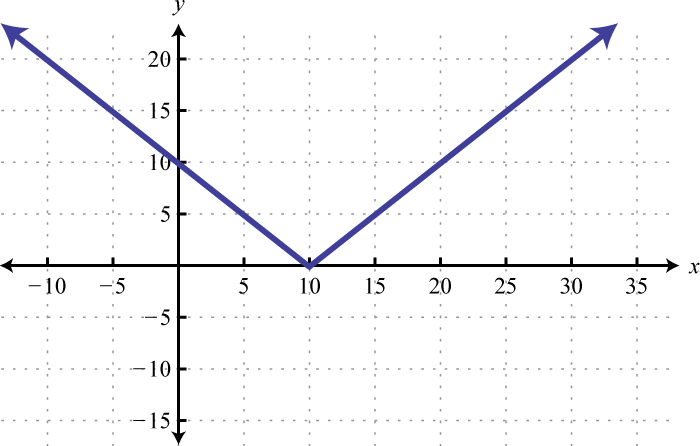3.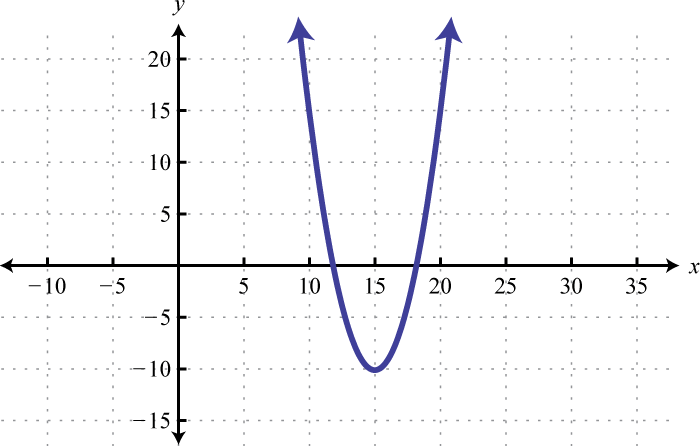4.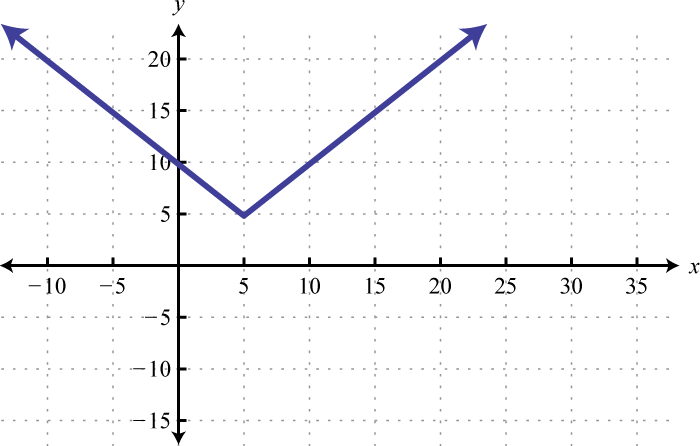5.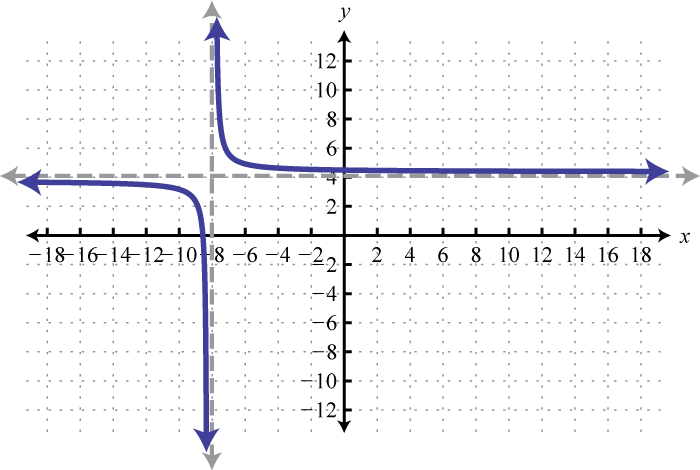6.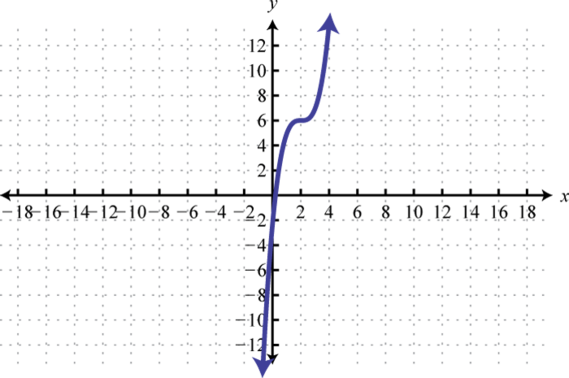7.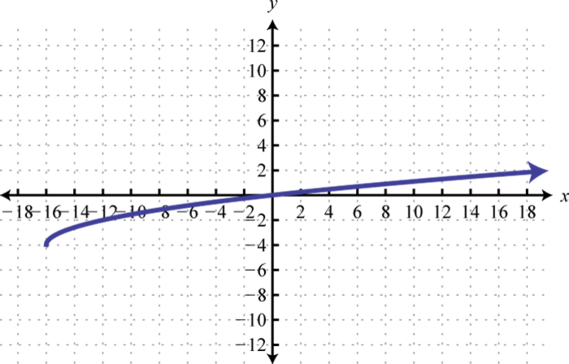8.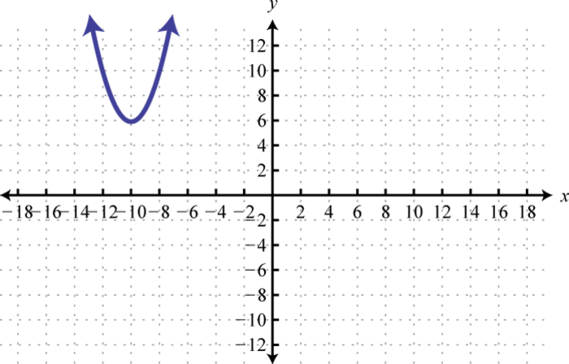### Part B: Reflections and Dilations

Match the graph the given function definition.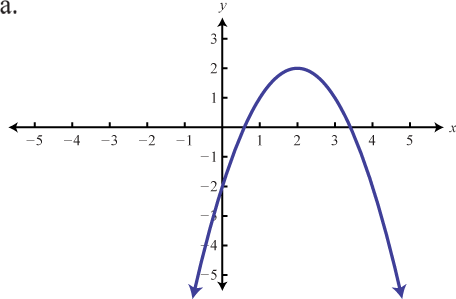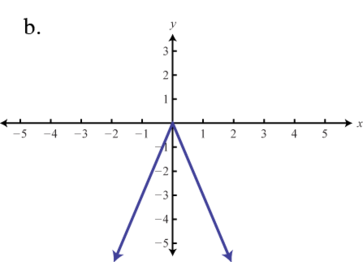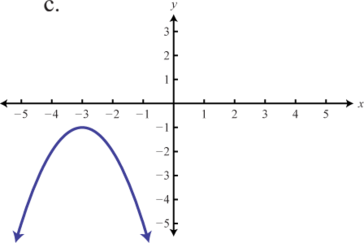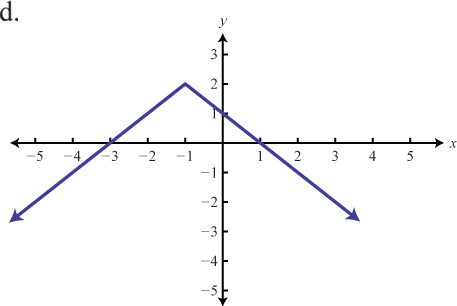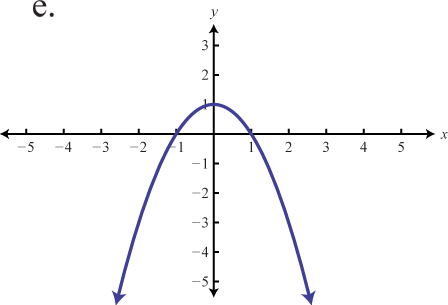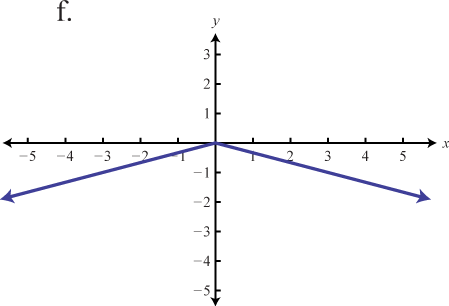1. $f(x)=−3|x|$

2. $f(x)=−(x+3)2−1$

3. $f(x)=−|x+1|+2$

4. $f(x)=−x2+1$

5. $f(x)=−13|x|$

6. $f(x)=−(x−2)2+2$

Use the transformations to graph the following functions.

1. $f(x)=−x+5$

2. $f(x)=−|x|−3$

3. $g(x)=−|x−1|$

4. $f(x)=−(x+2)2$

5. $h(x)=−x+2$

6. $g(x)=−x+2$

7. $g(x)=−(x+2)3$

8. $h(x)=−x−2+1$

9. $g(x)=−x3+4$

10. $f(x)=−x2+6$

11. $f(x)=−3|x|$

12. $g(x)=−2x2$

13. $h(x)=12(x−1)2$

14. $h(x)=13(x+2)2$

15. $g(x)=−12x−3$

16. $f(x)=−5x+2$

17. $f(x)=4x−1+2$

18. $h(x)=−2x+1$

19. $g(x)=−14(x+3)3−1$

20. $f(x)=−5(x−3)2+3$

21. $h(x)=−3|x+4|−2$

22. $f(x)=−1x$

23. $f(x)=−1x+2$

24. $f(x)=−1x+1+2$

### Part C: Discussion Board

1. Use different colors to graph the family of graphs defined by $y=kx2$, where $k∈{1,12,13,14}.$ What happens to the graph when the denominator of k is very large? Share your findings on the discussion board.

2. Graph $f(x)=x$ and $g(x)=−x$ on the same set of coordinate axes. What does the general shape look like? Try to find a single equation that describes the shape. Share your findings.

3. Explore what happens to the graph of a function when the domain values are multiplied by a factor a before the function is applied, $f(ax).$ Develop some rules for this situation and share them on the discussion board.

1. e

2. d

3. f

4.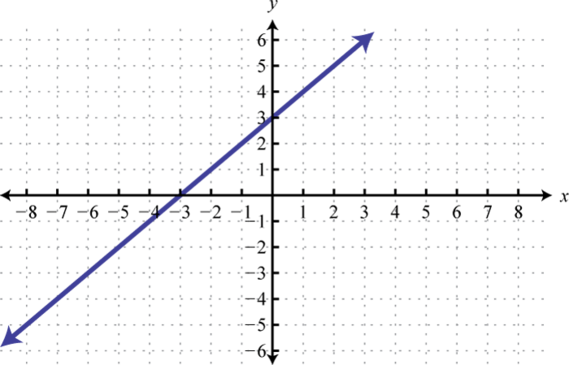$y=x$; Shift up 3 units; domain: $ℝ$; range: $ℝ$

5.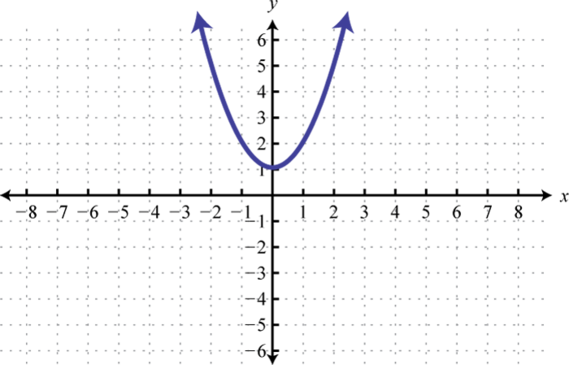$y=x2$; Shift up 1 unit; domain: $ℝ$; range: $[1,∞)$

6.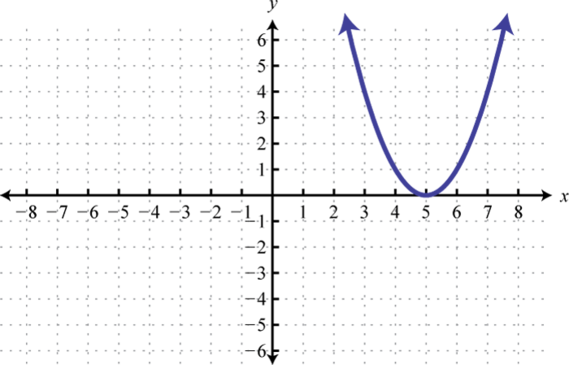$y=x2$; Shift right 5 units; domain: $ℝ$; range: $[0,∞)$

7.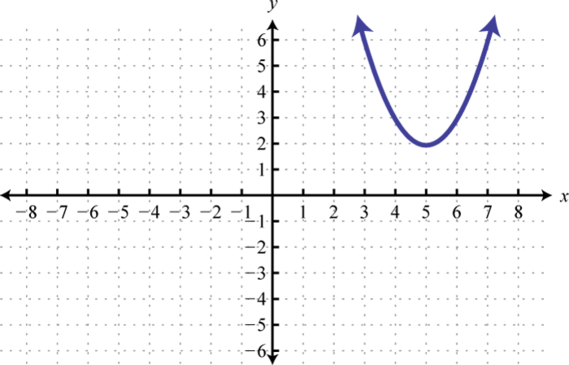$y=x2$; Shift right 5 units and up 2 units; domain: $ℝ$; range: $[2,∞)$

8.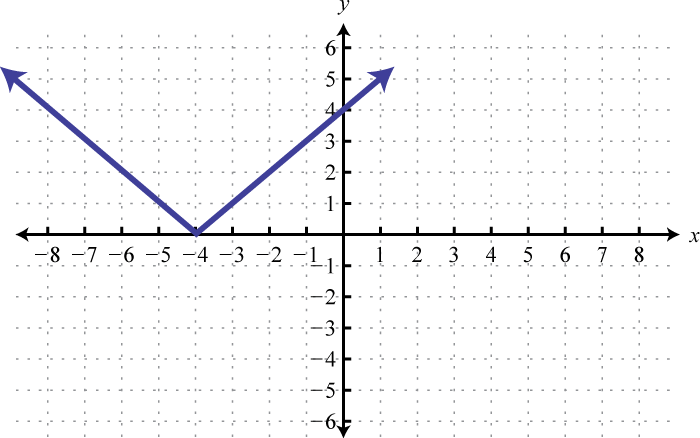$y=|x|$; Shift left 4 units; domain: $ℝ$; range: $[0,∞)$

9.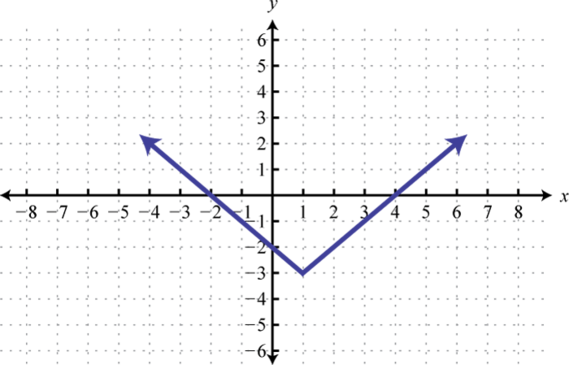$y=|x|$; Shift right 1 unit and down 3 units; domain: $ℝ$; range: $[−3,∞)$

10.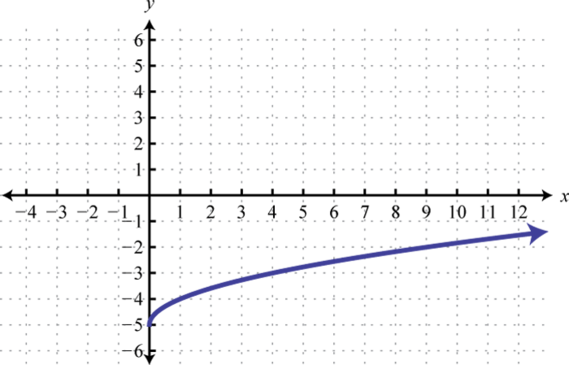$y=x$; Shift down 5 units; domain: $[0,∞)$; range: $[−5,∞)$

11.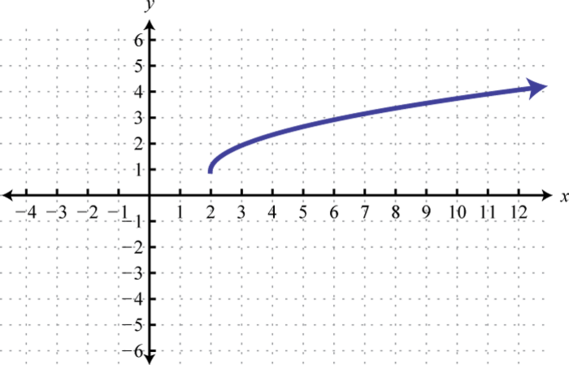$y=x$; Shift right 2 units and up 1 unit; domain: $[2,∞)$; range: $[1,∞)$

12.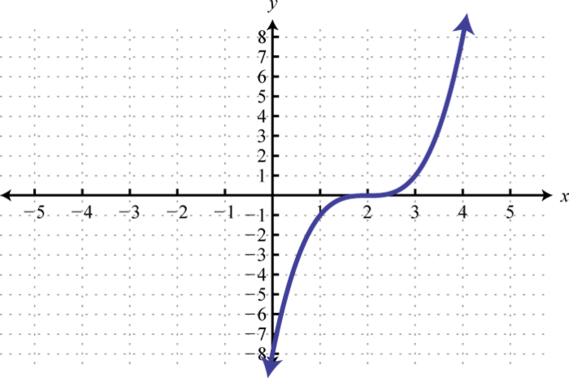$y=x3$; Shift right 2 units; domain: $ℝ$; range: $ℝ$

13.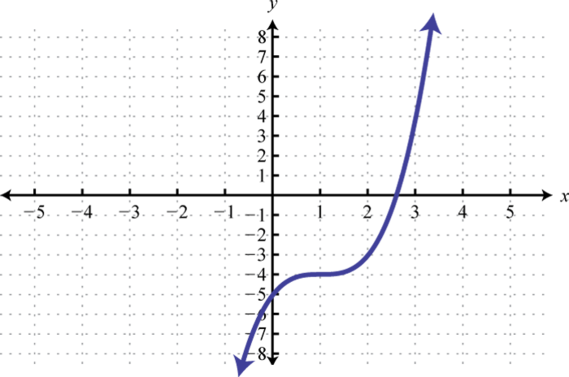$y=x3$; Shift right 1 unit and down 4 units; domain: $ℝ$; range: $ℝ$

14.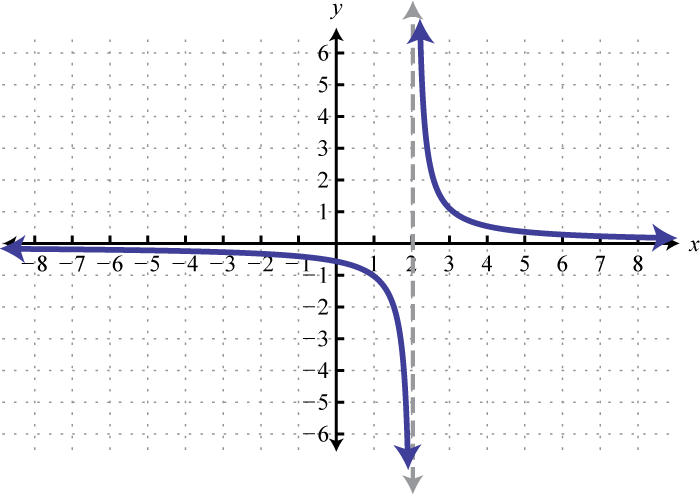$y=1x$; Shift right 2 units; domain: $(−∞,2)∪(2,∞)$; range: $(−∞,0)∪(0,∞)$

15.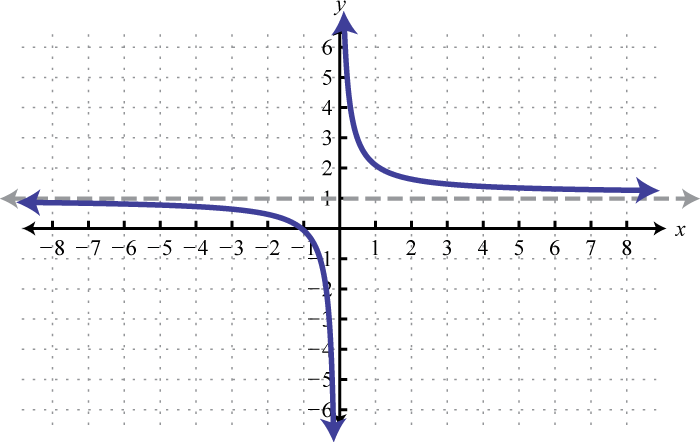$y=1x$; Shift up 5 units; domain: $(−∞,0)∪(0,∞)$; range: $(−∞,1)∪(1,∞)$

16.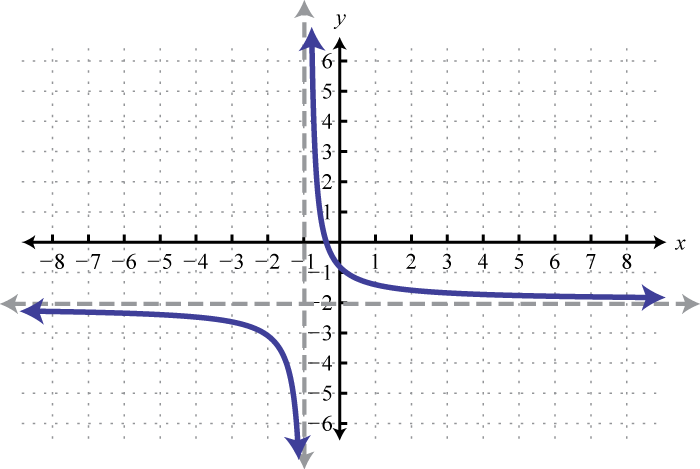$y=1x$; Shift left 1 unit and down 2 units; domain: $(−∞,−1)∪(−1,∞)$; range: $(−∞,−2)∪(−2,∞)$

17.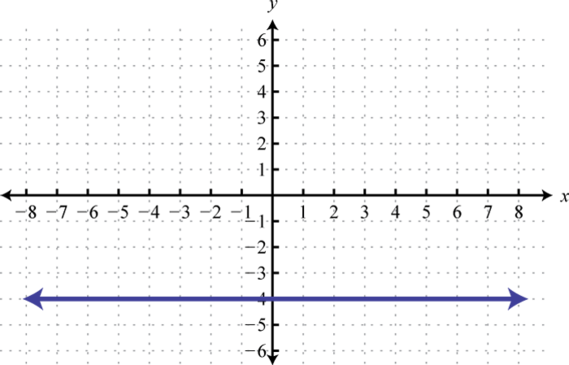Basic graph $y=−4$; domain: $ℝ$; range: {−4}

18.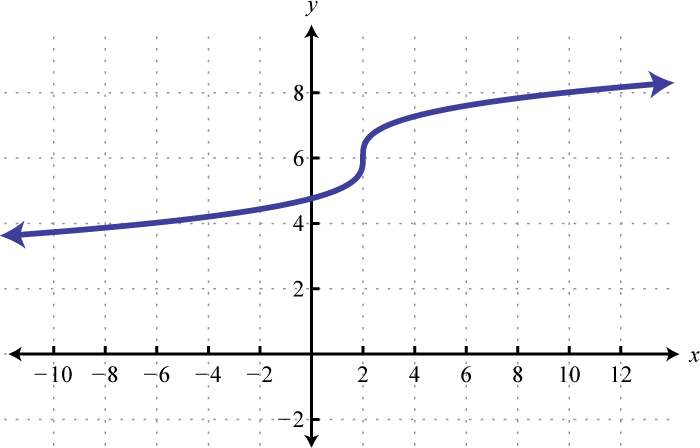$y=x3$; Shift up 6 units and right 2 units; domain: $ℝ$; range: $ℝ$

19.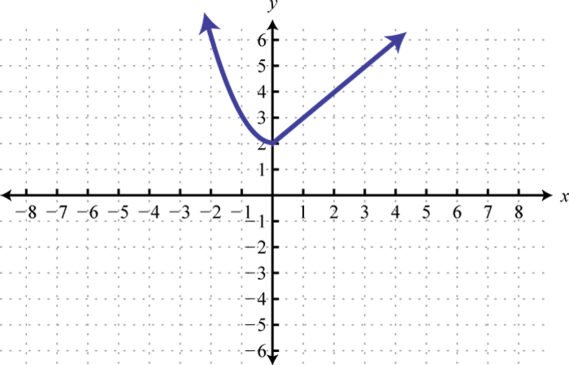20.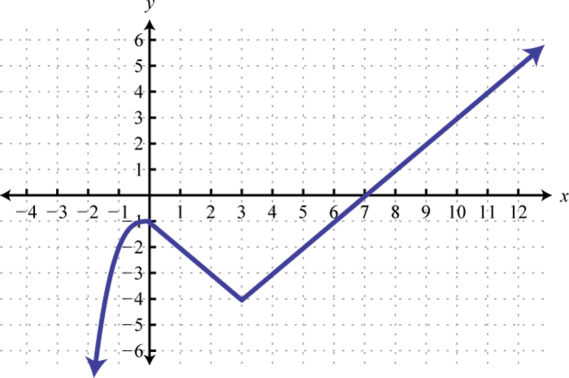21.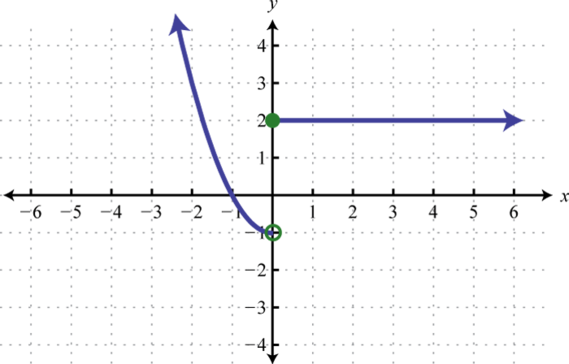22.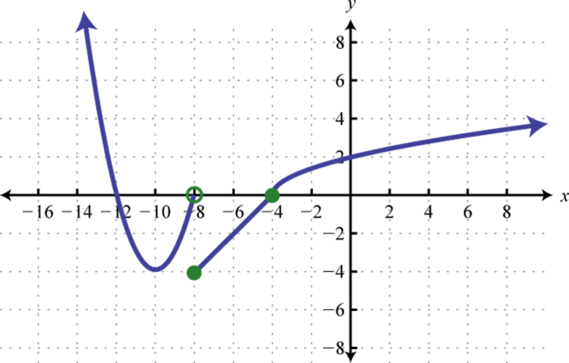23. $f(x)=x−5$

24. $f(x)=(x−15)2−10$

25. $f(x)=1x+8+4$

26. $f(x)=x+16−4$

1. b

2. d

3. f

4.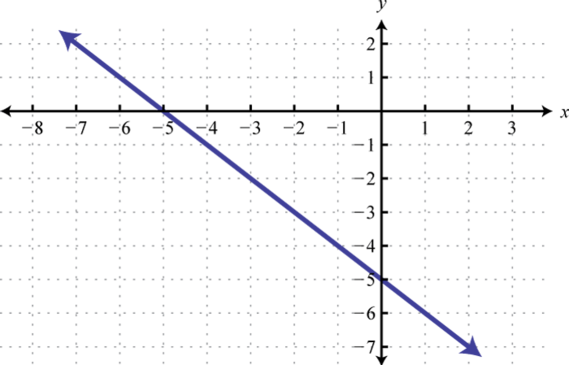5.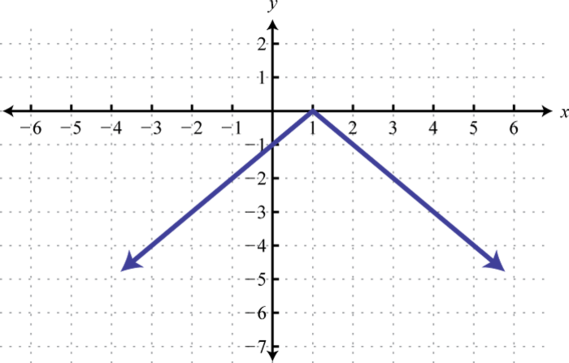6.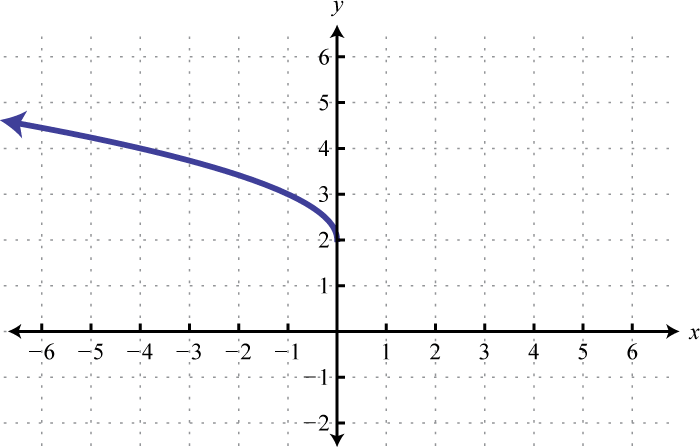7.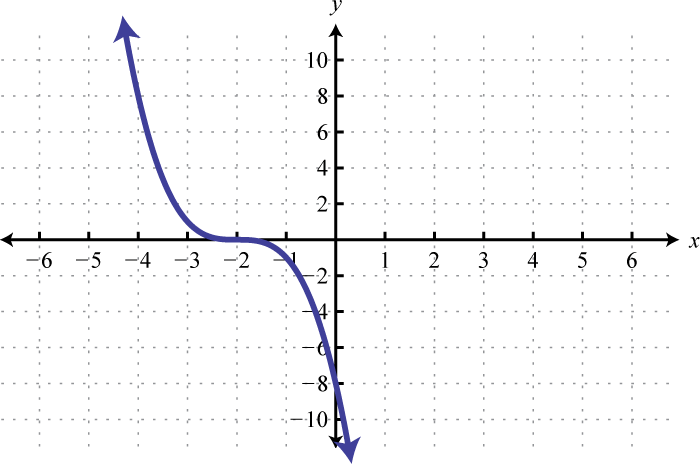8.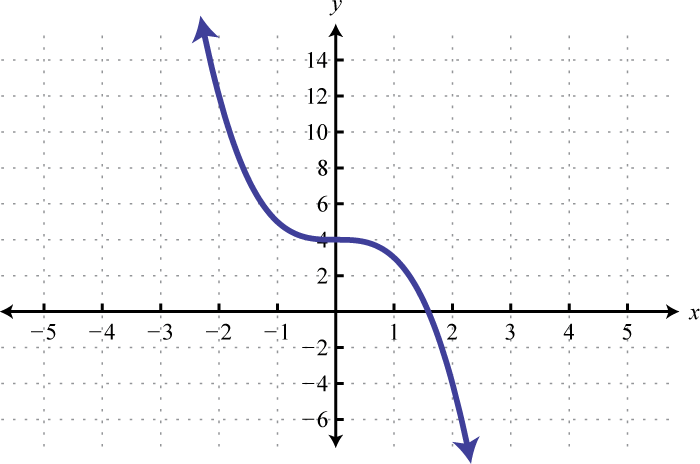9.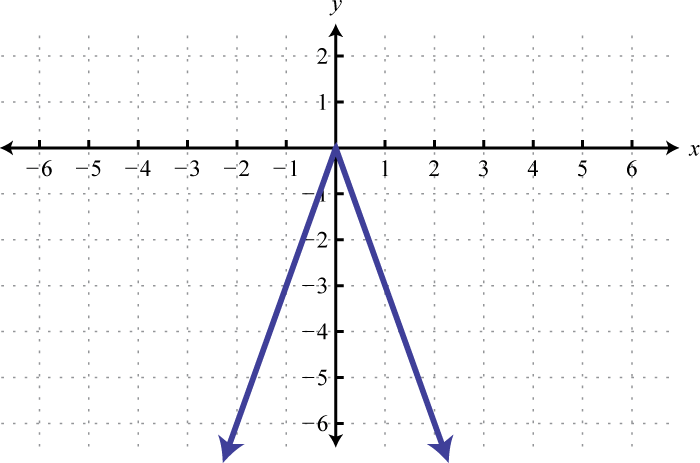10.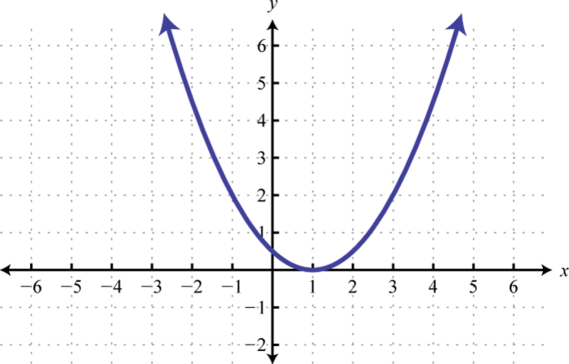11.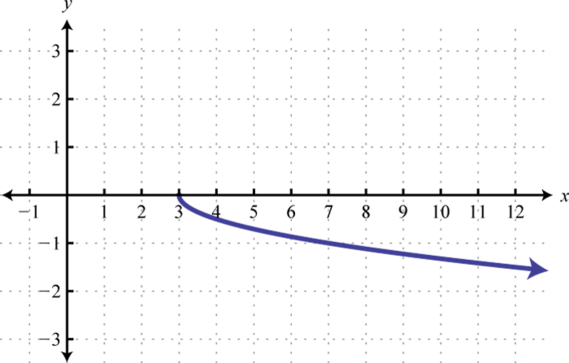12.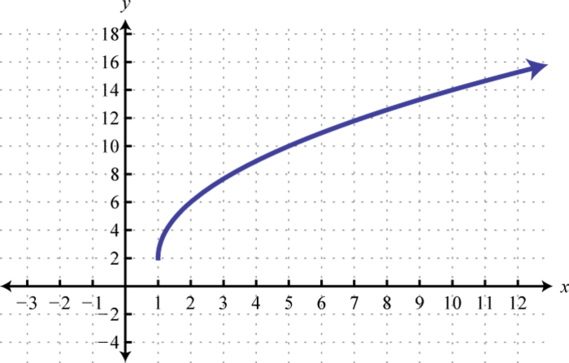13.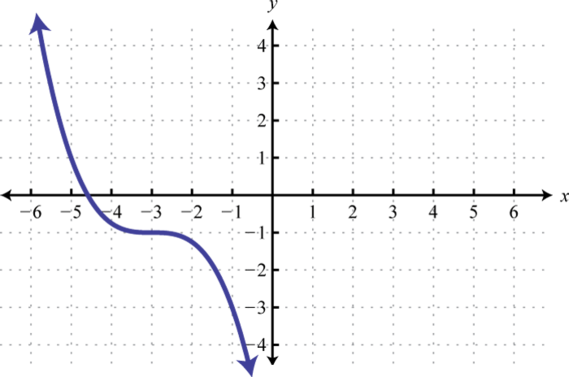14.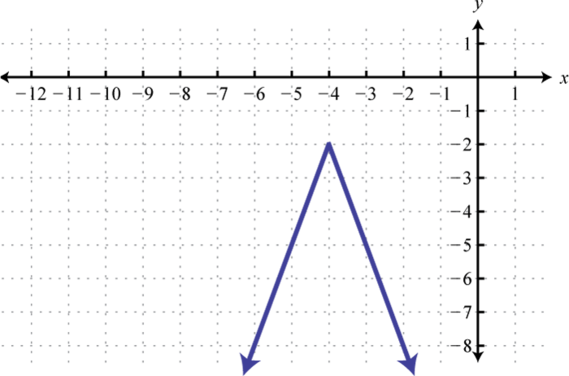15.